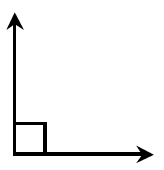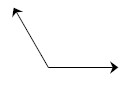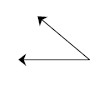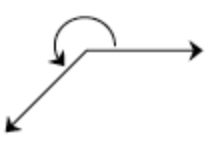Home > GC > Chapter 1 > Lesson 1.2.1 > Problem1-55

1-55.

Estimate the size of each angle below to the nearest $10°$. A right angle is shown at right for reference so you do not need a protractor. Homework Help ✎1.1.1.Look at problem 1-42. How do the angles relate to the right angle?

$120°$

$40°$

$230°$